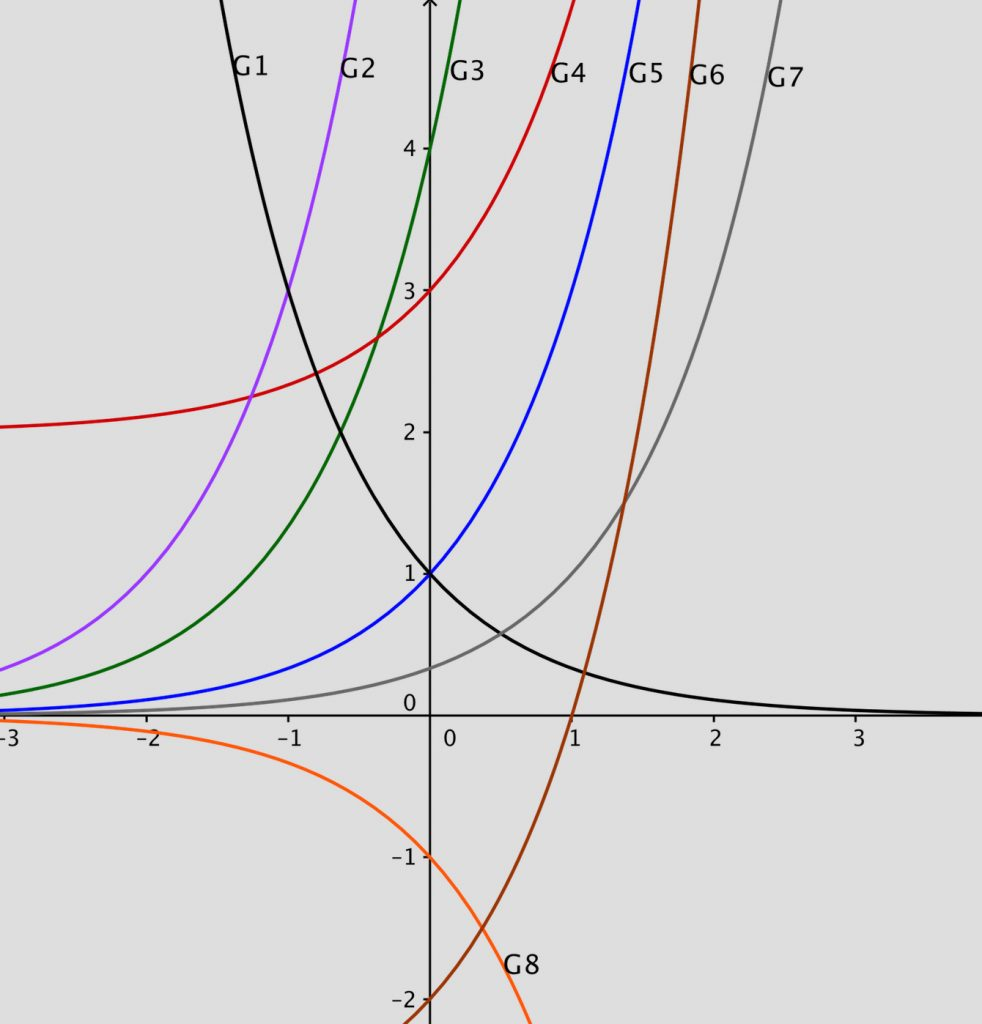The table below gives equations of the exponential functions shown in the graphs and descriptions of transformations, but jumbled up. Use your knowledge of transformations of functions to match the graphs  to their equations and to the descriptions of the transformations.

Cut up a copy of the table and rearrange the pieces into 8 matching sets.

 Equations Graphs Transformations 1 $$y=4.3^x$$ G1 A $$f(x)$$ shifted 3 units downwards 2 $$y=3^x+2$$ G2 B $$f(x)$$ was reflected in the axis 3 $$y=-3^x$$ G3 C $$f(x)$$ shifted 1 unit to the right 4 $$y=3^{x+2}$$ G4 D $$f(x)$$ shifted 2 units upwards 5 $$y=3^{x-1}$$ G5 E $$f(x)$$ was reflected in the axis 6 $$y=3^{-x}$$ G6 F $$f(x)$$ shifted 2 units to the left 7 $$y=3^x-3$$ G7 G $$f(x)$$ 8 $$y=3^x$$ G8 H $$f(x)$$ intersects y-axis at (0; 4)

Download a worksheet here for this activity.

Click here for Notes for Teachers.

### One Response to Grades 11 and 12 Matching Exponentials

1.MacDonald Chapwanya says:

I like the way latex integrates so smoothly with WordPress to produce these nice graphs and equations.

Set your Twitter account name in your settings to use the TwitterBar Section.